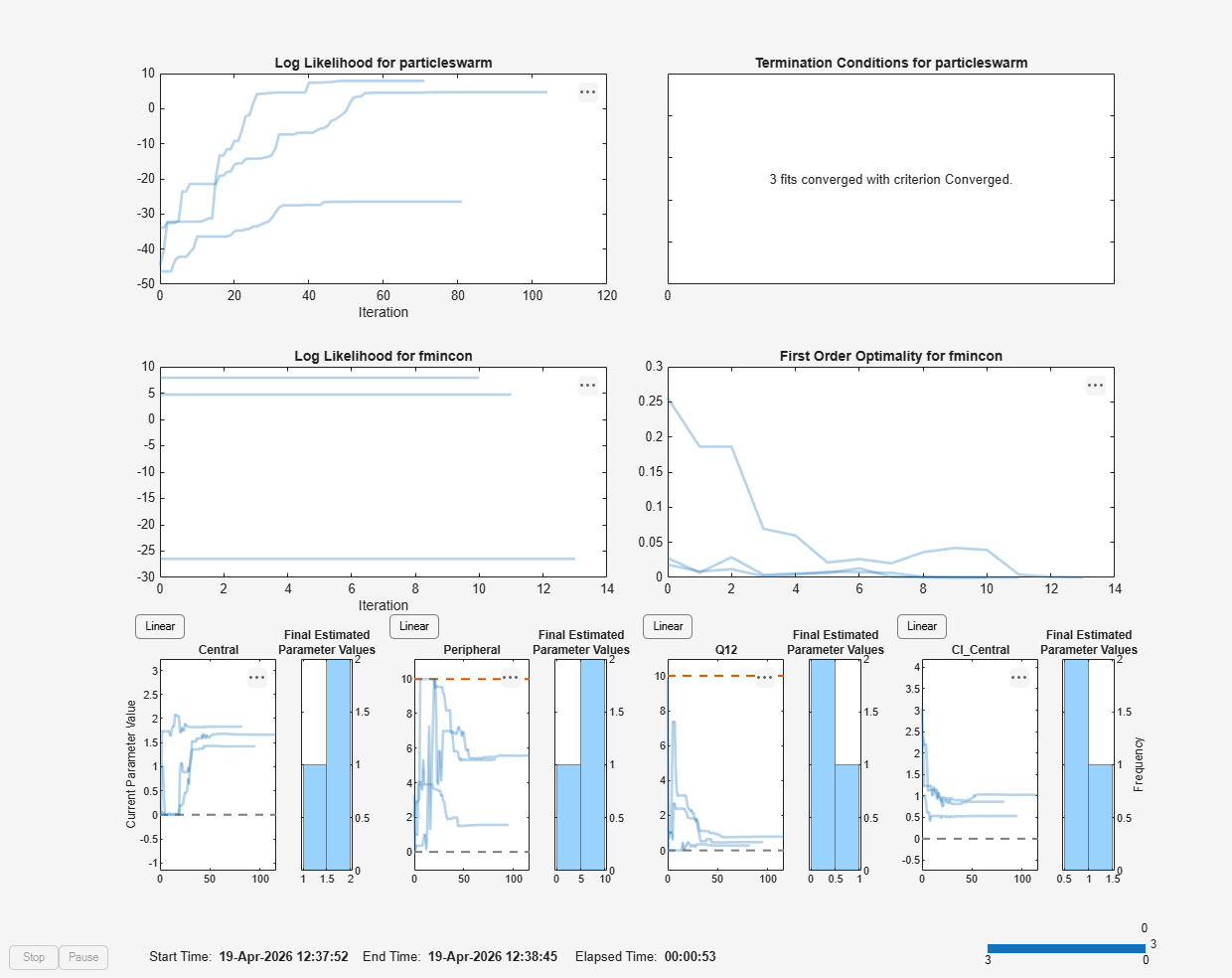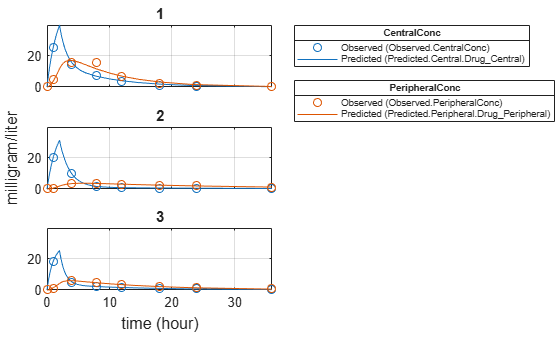Main Content

# Perform Hybrid Optimization Using sbiofit

This example shows how to configure `sbiofit` to perform a hybrid optimization by first running the global solver `particleswarm`, followed by another minimization function, `fmincon`.

### Load Data

Load the sample data to fit. The data is stored as a table with variables ID, Time, CentralConc, and PeripheralConc. This synthetic data represents the time course of plasma concentrations measured at eight different time points for both central and peripheral compartments after an infusion dose for three individuals. The dose amount is 100 milligram and dose rate is 50 milligram/hour.

```load('data10_32R.mat') gData = groupedData(data); gData.Properties.VariableUnits = {'','hour','milligram/liter','milligram/liter'}; sbiotrellis(gData,'ID','Time',{'CentralConc','PeripheralConc'},'Marker','+',... 'LineStyle','none');```### Create Model

Create a two-compartment model with an infusion dose.

```pkmd = PKModelDesign; pkc1 = addCompartment(pkmd,'Central'); pkc1.DosingType = 'Infusion'; pkc1.EliminationType = 'linear-clearance'; pkc1.HasResponseVariable = true; pkc2 = addCompartment(pkmd,'Peripheral'); model = construct(pkmd); configset = getconfigset(model); configset.CompileOptions.UnitConversion = true; dose = sbiodose('dose','TargetName','Drug_Central'); dose.StartTime = 0; dose.Amount = 100; dose.Rate = 50; dose.AmountUnits = 'milligram'; dose.TimeUnits = 'hour'; dose.RateUnits = 'milligram/hour'; responseMap = {'Drug_Central = CentralConc','Drug_Peripheral = PeripheralConc'};```

### Define Parameters to Estimate

Use the `estimatedInfo` object to define the estimated parameters.

```paramsToEstimate = {'log(Central)','log(Peripheral)','Q12','Cl_Central'}; estimatedParam = estimatedInfo(paramsToEstimate,'InitialValue',[1 1 1 1],... 'Bounds',[0 10]);```

### Define the Options for Hybrid Optimization

Define the options for the global solver and the hybrid solver. Because the parameters are bounded, make sure you use a compatible hybrid function for a constrained optimization, such as `fmincon`. Use `optimset` to define the options for `fminsearch`. Use `optimoptions` to define the options for `fminunc`, `patternsearch`, and `fmincon`.

```rng('default'); globalMethod = 'particleswarm'; options = optimoptions(globalMethod); hybridMethod = 'fmincon'; hybridopts = optimoptions(hybridMethod,'Display','none'); options = optimoptions(options,'HybridFcn',{hybridMethod,hybridopts});```

### Fit Data

Estimate model parameters. Turn on `ProgressPlot` to see the live feedback on the status of fitting. The first row of plots are the quality measure plots for the global solver. The second row plots are for the hybrid function. For details, see Progress Plot.

```unpooledFit = sbiofit(model,gData,responseMap,estimatedParam,dose,globalMethod,... options,'Pooled',false,'ProgressPlot',true);```### Plot Results

`plot(unpooledFit);`## SupportGet trial now